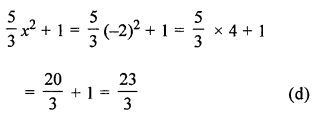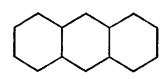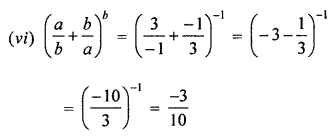## ML Aggarwal Class 7 Solutions for ICSE Maths Chapter 8 Algebraic Expressions Objective Type Questions

Mental Maths

Question 1.
Fill in the blanks:
(i) The terms with different algebraic factors are called ……….
(ii) The number of terms in a monomial is ………
(iii) An algebraic expression having two unlike terms is called a ……..
(iv) 3a2b and -7ba2 are ……….. terms.
(v) -6a2b and -6ab2 are ……… terms.
(vi) The number of unlike terms in the algebraic expression 3x2 – 2xy + 5x2 is ………
(vii) The factors of the term -3p2q2 are ……..
(viii) The perimeter of a triangle whose sides measure 2a, b and a + b is ………
(ix) The value of the expression 2x3 – 7x2 + 5x – 3 when x = 1 is ………..
(x) In the term -7a2bc, the coefficient of a is ……..
(xi) The degree of the polynomial 3 – 5x2 + 7x3 – x4 is ……….
(xii) The degree of the polynomial 3x2 – 2xy2 + 5 is ………
Solution:
(i) The terms with different algebraic factors
are called unlike terms.
(ii) The number of terms in a monomial is one.
(iii) An algebraic expression having two unlike terms
is called a bionomial.
(iv) 3a2b and -7ba2 are like terms.
(v) -6a2b and -6ab2 are unlike terms.
(vi) The number of unlike terms in the algebraic expression
3x2 – 2xy + 5x2 is 2.
(vii) The factors of the term -3p2q2 are -3, p, p, q, q.
(viii) The perimeter of a triangle whose sides measure
2a, b and a + b is 2a + b + a + b = 3a + 2b.
(ix) The value of the expression 2x3 – 7x2 + 5x – 3
when x = 1 is -3.
2(1)3 – 7(1)2 + 5(1) – 3
= 2 – 7 + 5 – 3
= -3
(x) In the term -7a2bc, the coefficient of a is -7abc.
(xi) The degree of the polynomial 3 – 5x2 + 7x3 – x4 is 4.
3 – 5x2 + 7x3 – x4 is 4
(xii) The degree of the polynomial 3x2 – 2xy2 + 5 is 3.
3x2 – 2xy2 + 5 is 1 + 2 = 3

Question 2.
State whether the following statements are true (T) or false (F).
(i) The expression 5x + 7 – 2x is a trinomial.
(ii) (7x – 10) – (3x – 5) = 4x – 15.
(iii) The coefficient of 3x in -3x3y is -xy.
(iv) The constant term in the expression 2x2 – 3xy – 7 is 7.
(v) If x = 3 and y = $$\frac { 1 }{ 3 }$$ then the value of xy (x2 + y2) is 9$$\frac { 1 }{ 9 }$$.
(vi) (3x – y + 5) – (x + y) is a binomial.
(vii) Sum of 2 and p is 2p.
(viii) Sum of x2 + x and y2 + y is 2x2 + 2y2.
(ix) In like terms, variables and their powers are the same.
(x) Every polynomial is a monomial.
(xi) If we add a monomial and a binomial, then answer can never be a monomial.
(xii) If we subtract a monomial from a binomial, then the answer is at least a binomial.
(xiii) if we add a monomial and a trinomial, then the answer can be a monomial.
(xiv) If we add a monomial and a binomial, then the answer can be a trinomial.
Solution:
(i) The expression 5x + 7 – 2x is a trinomial. (False)
Correct:
As 5x + 7 – 2x = 3x + 7 which has two terms.
(ii) (7x – 10) – (3x – 5) = 4x – 15. (False)
Correct:
(7x – 10) – (3x – 5) = 4x – 5
(iii) The coefficient of 3x in -3x3y is -xy. (False)
Correct:
As co-efficient of 3x is -x2y
(iv) The constant term in the expression
2x2 – 3xy – 7 is 7. (False)
Correct:
2x2 – 3xy – 7 is -7
(v) If x = 3 and y = $$\frac { 1 }{ 3 }$$
then the value of xy(x2 + y2) is 9$$\frac { 1 }{ 9 }$$. (True)(vi) (3x – y + 5) – (x + y) is a binomial. (False)
Correct:
= 3x – y + 5 – x + y
= 2x – 2y + 5
It is trinomial.
(vii) Sum of 2 and p is 2p. (False)
Correct:
Sum of 2 and p is 2 + p not 2p.
(viii) Sum of x2 + x and y2 + y is 2x2 + 2y2. (False)
Correct:
Sum of x2 + x and y2 + y = x2 + y2 + x + y not 2x2 + 2y2
(ix) In like terms, variables and their powers are same. (True)
(x) Every polynomial is a monomial. (False)
It could be binomial, trinomial or polynomial.
(xi) If we add a monomial and a binomial,
then answer can never be a monomial. (False)
Correct:
It can be monomial.
For example :
3x + (5 – 3x) = 3x + 5 – 3x = 5 which is monomial.
(xii) If we subtract a monomial from a binomial,
then the answer is at least a binomial. (False)
Correct:
It can be monomial also, for example
2x – (7 + 2x) = 2x – 2 – 2x = -7 which is a monomial.
(xiii) if we add a monomial and a trinomial,
then the answer can be a monomial. (False)
Correct:
It can be binomial also.
(xiv) If we add a monomial and a binomial,
then the answer can be a trinomial. (True)

Multiple Choice Questions

Choose the correct answer from the given four options (3 to 16):
Question 3.
The algebraic expression for the statement ‘Thrice square of a number x subtracted from five times the sum of y and 2’ is
(a) 5y + 2 – 3x2
(b) 3x2 – (5y + 2)
(c) 5(y + 2) – 3x2
(d) 5(y + 2) – (3x)2
Solution:
For the statement, thrice square of a number x
subtracted from five times the sum of y and 2 is 5(y + 2) – 3x2 (c)

Question 4.
The expression 7x – 5(x2 + y2) is a
(a) monomial
(b) binomial
(c) trinomial
(d) none of these
Solution:
7x – 5(x2 + y2) = 7x – 5x2 – 5y2
It is trinomial. (c)

Question 5.
The coefficient of 5a2 in -5a3bc is
(a) -bc
(b) a2bc
(c) -a2bc
(d) -abc
Solution:
Co-efficient of 5a2 in -5a3bc is -abc (d)

Question 6.
Which of the following is a pair of like terms?
(a) -5xy, 5x
(b) -5xy, 3yz
(c) -5xy, -5y
(d) -5xy, 7yx
Solution:
-5xy, 7yx is a pair of like terms. (d)

Question 7.
The like terms is the expressions 3x(3 – 2y) and 2(xy + x2) are
(a) 9x and 2x2
(b) -6xy and 2xy
(c) 9x and 2xy
(d) -6xy and 2x2
Solution:
Like terms in the expression
3x(3 – 2y) = 9x – 6xy and 2(xy + x2) = 2xy + 2x2
are -6xy and 2xy (b)

Question 8.
Identify the binomial out of the following:
(a) 3xy2 + 5y – x2y
(b) 2x2y – 5y – 2x2y
(c) 3xy2 + 5y – xy2
(d) xy + yz + zx
Solution:
(a) 3xy2 + 5y – x2y trinomial
(b) 2x2y – 5y – 2x2y = -5y monomial
(c) 3xy2 + 5y – xy2 = 2xy2 + 5y binomial (c)

Question 9.
The number of (unlike) terms in the expression 3xy2 + 2y2z – y2x + y(xz + yz) – 5
(a) 3
(b) 4
(c) 5
(d) 6
Solution:
The number of unlike terms in the expression
= 3xy2 + 2y2z – y2x + y(xz + yz) – 5
= 3xy2 + 2y2z – y2x + xyz + y2z – 5
= 2xy2 + 3y2z2 + xyz – 5
= 4 (b)

Question 10.
The value of the expression x3 + y3 when x = 2 and y = -2 is
(a) 0
(b) 8
(c) 16
(d) -16
Solution:
Value of x3 + y3 = (2)3 + (-2)3 = 8 – 8 = 0 (a)

Question 11.
-xy – (-5xy) is equal to
(a) -6xy
(b) 6xy
(c) -4xy
(d) 4xy
Solution:
-xy – (-5xy) = -xy + 5xy = 4xy (d)

Question 12.
On subtracting 7x + 5y – 3 from 5y – 3x – 9, we get
(a) 10x + 6
(b) -10x – 6
(c) 10x + 10y – 12
(d) -10x – 12
Solution:
(5y – 3x – 9) – (7x + 5y – 3)
= 5y – 3x – 9 – 7x – 5y + 3
= -10x – 6 (b)

Question 13.
The value of the expression $$\frac { 5 }{ 3 }$$ x2 + 1 when x = -2 is
(a) $$\frac { -17 }{ 3 }$$
(b) $$\frac { -7 }{ 3 }$$
(c) $$\frac { 21 }{ 3 }$$
(d) $$\frac { 23 }{ 3 }$$
Solution:Question 14.
The number of sides in a pattern having 3 hexagons arranged in a row as shown in the given figure is(a) 18
(b) 17
(c) 16
(d) 15
Solution:
Number of sides are = 16 (c)

Question 15.
The degree of the polynomial 3x3y – 5xy4 – 2x + 1 is
(a) 5
(b) 4
(c) 3
(d) 2
Solution:
The degree of the polynomial
3x3y – 5xy4 – 2x + 1 is of
-5xy4 = 1 + 4 = 5 (a)

Higher Order Thinking Skills (HOTS)

Question 1.
The length of a rectangle is 3x – 4y + 6z and the perimeter is 7x + 8y + 17z, find the breadth of the rectangle.
Solution:
Length of rectangle = 3x – 4y + 6z
2 × length = 6x – 8y + 12z
and perimeter = 7x + 8y + 17z = 2(Length + Breadth)
2 × Breadth = 7x + 8y + 17z – 6x + 8y – 12z = x + 5 z + 16y
Breadth = $$\frac { x }{ 2 }$$ + 8y + $$\frac { 5 }{ 2 }$$ z

Question 2.Solution:Question 3.
If a = 3, b = -1, then find the value of each of the following:Solution: